## Cube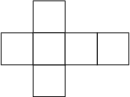The three-dimensional Platonic Solidwhich is also called the Hexahedron. The cube is composed of six Square faces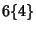which meet each other at Right Angles, and has 8 Vertices and 12 Edges. It is described by the Schläfli Symbol. It is a Zonohedron. It is also the Uniform Polyhedronwith Wythoff Symbol. It has the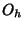Octahedral Group of symmetries. The Dual Polyhedron of the cube is the Octahedron.

Because the Volume of a cube of side lengthis given by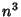, a number of the formis called a Cubic Number (or sometimes simply a cube''). Similarly, the operation of taking a number to the third Power is called Cubing.

The cube cannot be Stellated. A Plane passing through the Midpoints of opposite sides (perpendicular to aaxis) cuts the cube in a regular Hexagonal Cross-Section (Gardner 1960; Steinhaus 1983, p. 170; Cundy and Rollett 1989, p. 157; Holden 1991, pp. 22-23). Since there are four such axes, there are four possible hexagonal cross-sections. If the vertices of the cube are, then the vertices of the inscribed Hexagon are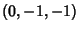,,,,, and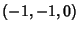. The largest Square which will fit inside a cube of sidehas each corner a distance 1/4 from a corner of a cube. The resulting Square has side length, and the cube containing that side is called Prince Rupert's Cube.

The solid formed by the faces having the sides of the Stella Octangula (left figure) as Diagonals is a cube (right figure; Ball and Coxeter 1987).

The Vertices of a cube of side length 2 with face-centered axes are given by. If the cube is oriented with a space diagonal along the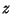-axis, the coordinates are (0, 0,), (0,,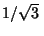), (,,), (,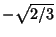,), (0,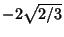,), (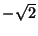,,), (,,), and the negatives of these vectors. A Faceted version is the Great Cubicuboctahedron.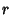(1)(2)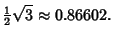(3)

The cube has a Dihedral Angle of(4)

The Surface Area and Volume of the cube are(5)(6)

See also Augmented Truncated Cube, Biaugmented Truncated Cube, Bidiakis Cube, Bislit Cube, Browkin's Theorem, Cube Dissection, Cube Dovetailing Problem, Cube Duplication, Cubic Number, Cubical Graph, Hadwiger Problem, Hypercube, Keller's Conjecture, Prince Rupert's Cube, Rubik's Cube, Soma Cube, Stella Octangula, Tesseract

References

Beyer, W. H. (Ed.) CRC Standard Mathematical Tables, 28th ed. Boca Raton, FL: CRC Press, p. 127, 1987.

Cundy, H. and Rollett, A. Hexagonal Section of a Cube.'' §3.15.1 in Mathematical Models, 3rd ed. Stradbroke, England: Tarquin Pub., p. 157, 1989.

Davie, T. The Cube (Hexahedron).'' http://www.dcs.st-and.ac.uk/~ad/mathrecs/polyhedra/cube.html.

Eppstein, D. Rectilinear Geometry.'' http://www.ics.uci.edu/~eppstein/junkyard/rect.html.

Gardner, M. Mathematical Games: More About the Shapes that Can Be Made with Complex Dominoes.'' Sci. Amer. 203, 186-198, Nov. 1960.

Holden, A. Shapes, Space, and Symmetry. New York: Dover, 1991.

Steinhaus, H. Mathematical Snapshots, 3rd American ed. New York: Oxford University Press, 1983.